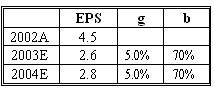### CFA Practice Question

There are 96 practice questions for this study session.

### CFA Practice Question

An analyst decides to calculate a stock's leading and trailing P/E ratios justified by fundamentals and determine the company's relative valuation based on its actual P/E ratio. The current year is 2002 and the current stock price is 32. The required rate of return is 10%.Based on the data, the analyst should conclude that:
A. The stock is overvalued.
B. The stock is undervalued.
C. The trailing and leading P/Es produce conflicting results.
Explanation: Fundamentals-based Trailing P/E = (1- b) (1 + g) / (r - g) = 0.3 x (1 + 0.05) / (0.10 - 0.05) = 6.3.

Fundamentals-based Leading P/E = (1 - b) / (r - g) = 0.3 / (0.10 - 0.05) = 6.

Actual Trailing P/E = P2002/E2002 = 32/4.5 = 7.11.

Actual Leading P/E = P2002/E2003= 32/2.6 = 12.31.

Both trailing and leading P/Es based on fundamentals are lower than the actual P/Es, respectively. This means that the company may be overvalued.